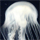## General Question# If A and B are events such that ​P(A) =0.6 and ​P(AU​B)​ =.08, find​ P(B) when ​(a) A and B are​ disjoint; ​(b) A and B are independent.

Asked by MrMikeyKombat07 (13) September 28th, 2020

So I understand the 1st part of the question and I know that you take “A” and subtract 0.6 from 0.8 which gives me 0.2. I’m stuck on part B and don’t know what I’m messing up on. Anyways, thank you for all the help!

Observing members: 0Composing members: 0We know that for independent events A and B, P (A and B) = P(A)P(B), which in this case is .6x.8=.48

Here are two equivalent ways of using this.

1. To get three disjoint probabilities, subtract the probability of (A and B) from both A and B, giving probabilities of (.6 – .48), (,8 – .48) and .48. Now just add these to together.

2. If you add the probabilities of A and B, the probability of (A and B) will be counted twice, so to get the probability of (AUB), subtract probability of (A and B) from the sum to get .6 + .8 – .48

LostInParadise (29005)“Great Answer” (3) Flag as…Note that I used .8 instead of .08, so replace .48 with .048 in the above equations.

LostInParadise (29005)“Great Answer” (2) Flag as…Awesome thank you very much! This stuff can be a little tricky and it’s pretty new to me still so thank you for clearing this up!

MrMikeyKombat07 (13)“Great Answer” (1) Flag as…Thanks for the kind words. It may help to visualize if you think about Venn diagrams. A and B are two circles. AUB is the total area of the two circles and (A and B) is the area where they overlap.

LostInParadise (29005)“Great Answer” (1) Flag as…or